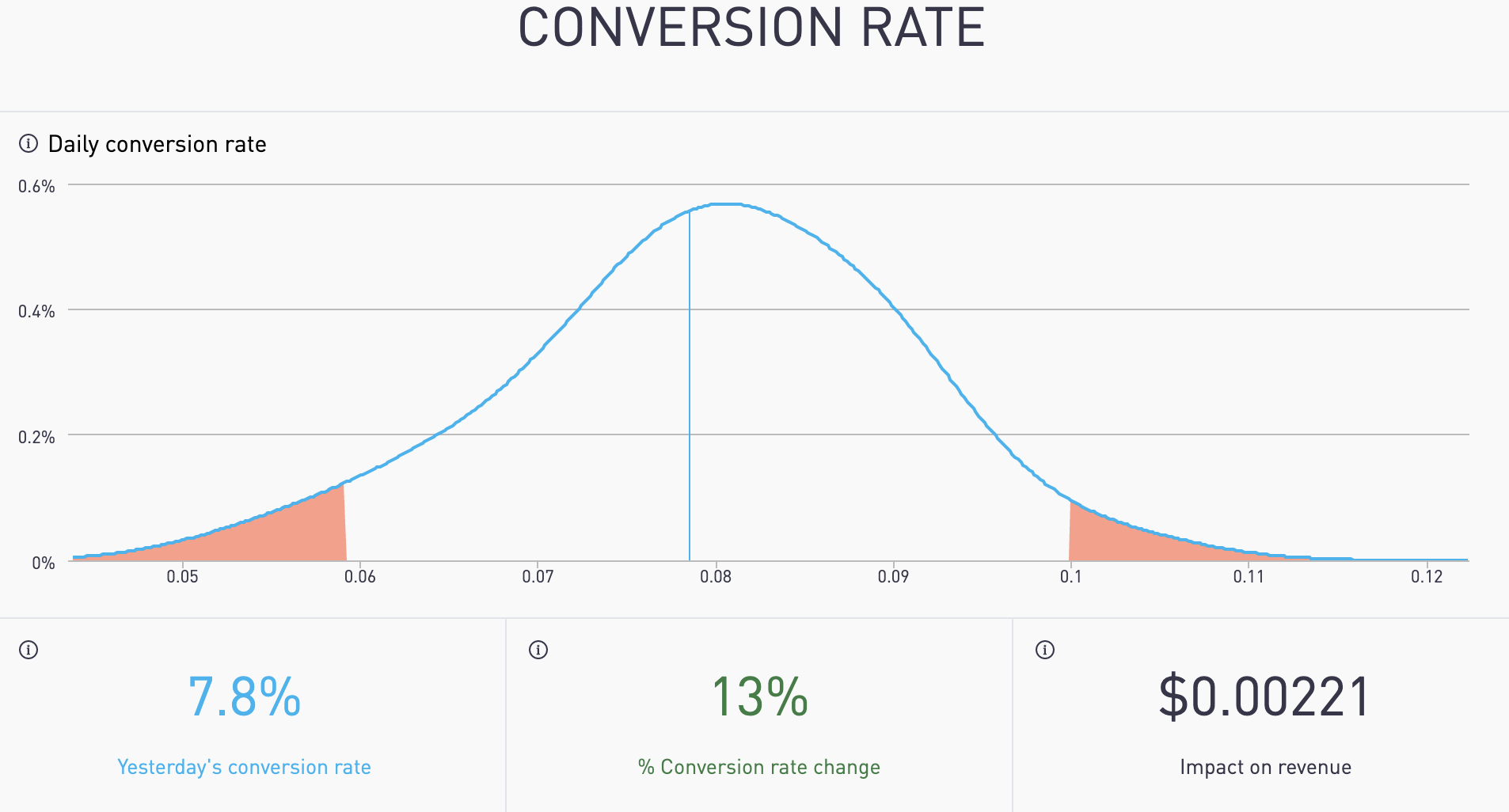# Conversion Rate

"Do I need to do something about my conversion rate?"

See yesterday's conversion rate. What impact did it have on your revenue?## Is it today's result common? Is it rare?

Density charts help you to see beyond simple averages to know if you need to take action. Decision-makers within companies have to focus on what matters. A simple average can't tell you when something is unusually good or bad. The density chart tells you how often something happens.

(density charts to detect trends) A density chart is the distribution of data over a continuous interval or time period.

# The Terms

Yesterday's Conversion Rate

The number of purchases divided by the number of shoppers for yesterday.

% Conversion Rate Change

The change in yesterday's conversion rate from the average of the last 4 weeks same day as yesterday conversion rate as a percentage.

Impact on Revenue

The impact change in conversion rate has on total revenue.

# The Math

Yesterday's Conversion Rate

Total sales by count divided by total visitors to the payment page for yesterday.

% Conversion Rate Change

If yesterday was "Sunday", then the yesterday conversion rate is compared with the average of previous 4 "Sunday's conversion rate and represented as a percentage.

Impact on Revenue

Calculated by finding the regression coefficient for conversion rate using linear regression multiplied with the change in conversion rate(Eg-The difference between Sunday's conversion rate & the previous 4 Sunday's conversion rate multiplied with the regression coefficient), represented as a US dollar amount.

# The Visuals

X axis (or horizontal axis)

Conversion rate

Y axis (or vertical axis)

How often a particular conversion rate occurred during the period. This is also known as frequency.

Blue Curved Line

The frequency of each conversion rate during the period shown on a smoothed line.

Blue Vertical Line

Yesterday's conversion rate.